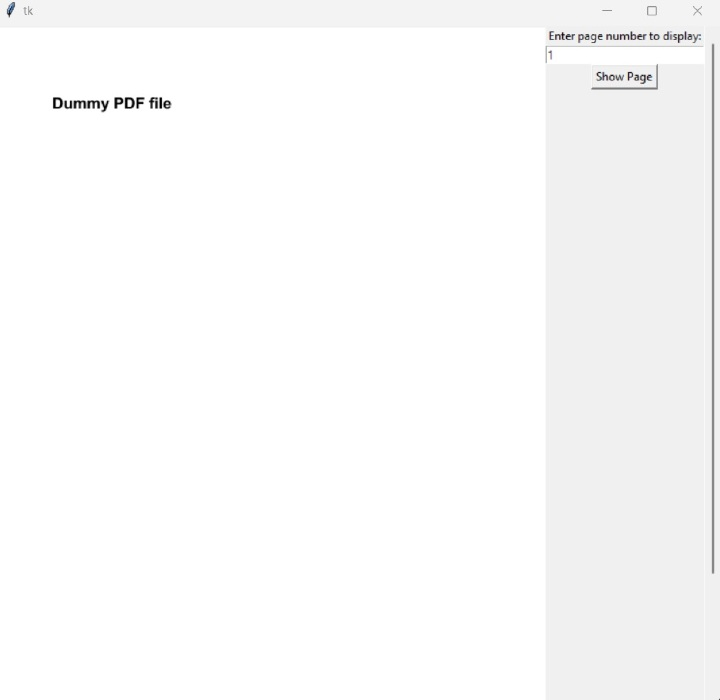# How to add PDF in Tkinter GUI Python?

This article will teach us how to display PDF files in the Tkinter GUI. We will be using the PyMuPDF library to read the pdf files and convert them into images which will then be displayed using Tkinter.

For our task, we will do the following steps −

• Define a transformation matrix to apply on the pages of PDF to get their images.

• Count the total number of pages for error checking.

• Define the screen (the canvas) for our GUI.

• Define a helper function for converting a PDF page to a PIL image.

• Define a function to show the image of a page in our GUI.

• Add buttons, labels, scrollbars etc and configure them.

To get started, let's first install the PyMuPDF package by running the following command in our terminal.

python -m pip install --upgrade pip
python -m pip install --upgrade pymupdf


Now we need to import the libraries we will be working with. import fitz

import fitz
from tkinter import *
from PIL import Image, ImageTk


Here fitz is a utility library that comes along with the PyMuPDF library and is used to get PDF pages as images.

We first open the PDF file we want to display in our GUI by using the fitz.open function. This returns a document object which can be used to access pages.

# open pdf file
file_name = "sample.pdf"
doc = fitz.open(file_name)


Now we need to specify how we would like to view the pages. By defining the below matrix function we are fitz to keep a 1x zoom.

# transformation matrix we can apply on pages
zoom = 1
mat = fitz.Matrix(zoom, zoom)


Let us also count the number of pages −

# count number of pages
num_pages = 0
for p in doc:
num_pages += 1


Now we will define a screen, add a scroll bar and a canvas to our code −

# initialize and set screen size
root = Tk()
root.geometry('750x700')

scrollbar = Scrollbar(root)
scrollbar.pack(side = RIGHT, fill = Y)

canvas = Canvas(root, yscrollcommand = scrollbar.set)
canvas.pack(side = LEFT, fill = BOTH, expand = 1)


Here our screen is represented by the name “root” and is of dimensions 750x700. We have also added a scroll bar and specified it to be on the right side and fill the entire height. Similarly, we have added the canvas to the left side and fill the entire screen on both sides!

In order to receive the page number the user wants to read, we need to take the page number as an input. We can do it as follows −

# define entry point (field for taking inputs)
entry = Entry(root)

# add a label for the entry point
label = Label(root, text="Enter page number to display:")


The arguments ‘root’ tell us that the objects will be associated with the screen “root”.

Now we will define a helper function to get the image of the PDF file from a page number.

def pdf_to_img(page_num):
pix = page.get_pixmap(matrix=mat)
return Image.frombytes("RGB", [pix.width, pix.height], pix.samples)


Here we first load the page using doc.load_page and passing the page number as an argument. Then we convert the PDF page to get pixel representation of that page in the second line. Finally, we convert the pixel representation into the PIL Image format.

Now we define a function show_image to display the image in our Tkinter GUI.

def show_image():
try:
page_num = int(entry.get()) - 1
assert page_num >= 0 and page_num < num_pages
im = pdf_to_img(page_num)
img_tk = ImageTk.PhotoImage(im)
frame = Frame(canvas)
panel = Label(frame, image=img_tk)
panel.pack(side="bottom", fill="both", expand="yes")
frame.image = img_tk
canvas.create_window(0, 0, anchor='nw', window=frame)
canvas.config(scrollregion=canvas.bbox("all"))
except:
pass


Here first we read the page number from the entry point we defined earlier and convert it to an integer. If it’s not a valid integer, an exception occurs and the exception block handles it. We then check if the page number is between 0 or the number of pages since that is the only valid range of pages we can display. Then using our previously defined helper function, we get a PIL Image of the user specified page. We then set the frame, panel and the canvas to appropriately display this image.

Next we add a button to display the page. We pass the function show_image as a command. Hence whenever the button is pressed the show_image function will be called.

# add button to display pages
button = Button(root, text="Show Page", command=show_image)


Now we set the visual locations of the various components we defined previously −

# set visual locations
label.pack(side=TOP, fill=None)
entry.pack(side=TOP, fill=BOTH)
button.pack(side=TOP, fill=None)


We also set the initial page as the first page of the PDF as follows −

entry.insert(0, '1')
show_image()


We configure the scroll bar

scrollbar.config(command = canvas.yview)
root.mainloop()


Finally we close the PDF so it doesn’t get corrupted by our computer.

doc.close()


## Output

After running the program we see the following output!## Conclusion

This article taught us how to use the PyMuPDF library along with Pillow and Tkinter to display a PDF file in the Tkinter GUI. We also saw how to count the number of pages, convert a PDF page to an image, make interactive buttons and how to visually place different elements that make up our GUI.

Updated on: 23-Mar-2023

1K+ Views